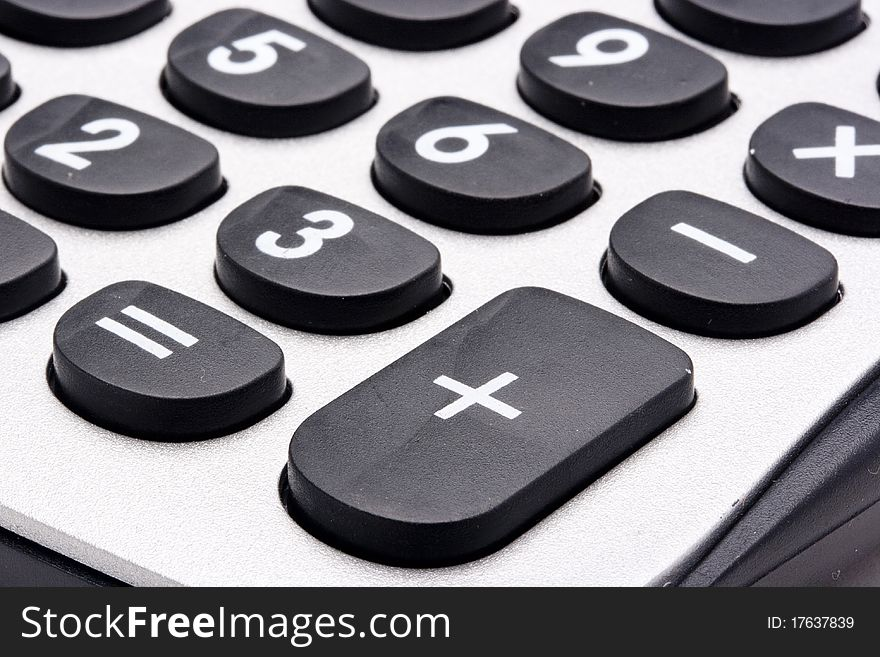DismissalForm For TaxCalculatorCalculator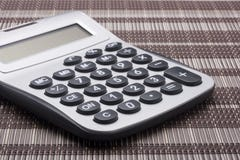Calculator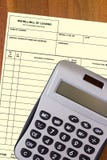Calculator With A Blank Waybill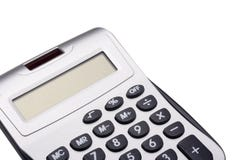Calculator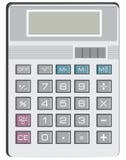Calculator For Simple Arithmetic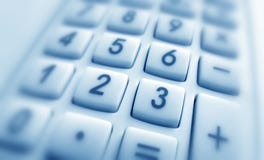CalculatorCalculator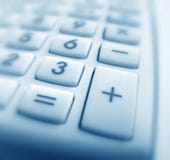CalculatorCalculator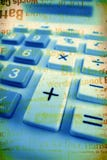CalculatorCalculatorCalculator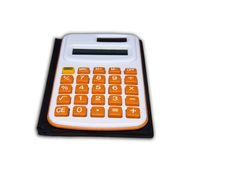CalculatorBusinessman Showing Calculator With 1000+Businessman With Gigantic Pocket Calculator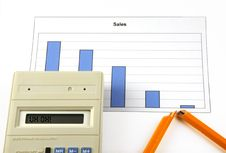Calculator With Text Uh Oh!, Graph And Broken PenMan With Calculator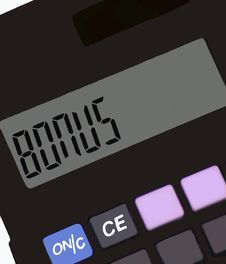CalculatorCalculator With Hand